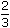# Aptitude - Stocks and Shares - Discussion

Discussion Forum : Stocks and Shares - General Questions (Q.No. 5)
5.
By investing in 16% stock at 64, one earns Rs. 1500. The investment made is:
Rs. 5640
Rs. 5760
Rs. 7500
Rs. 9600
Explanation:

 To earn Rs. 50 , investment = Rs. 64. 3

 To earn Rs. 1500, investment = Rs.64 x 3 x 1500= Rs. 5760. 50

Discussion:
48 comments Page 1 of 5.

Raam said:   4 months ago
@Dhivya.

How did you compare 50/3x = 64*1500.
you mentioned in the above equation that;
50/3*64.

1500*x
therefore,50/3*64 =1500*x,
this might be possible.
Then how did you declared that comparison? Please explain to me.

Dhivya said:   8 months ago
@All.

Solve it by logic;

For earning rs.50/3 he has invested Rs.64.
To earn Rs.1500 how much thus he had to invest,
50/3. 64.
1500. x.

50/3x = 64 * 1500.
Therefore:
x = 64 * 1500 * 3/50.
Ans : 5760.

Hope this might help.
(1)

Roshni said:   2 years ago
Isn't is 50/3 when investing 100?

So the profit when 64 is invested is 64*50/300?

Shivansh said:   2 years ago
Let's say person earn X% When he invest Rs 64 which comes out to be Rs 50/3
64 * x% = 50/3.
Now you can find x value correct which is 3.8% so now find 38% of 1500= 5760.

Shubham said:   3 years ago
The profit is 50/3 on investing 64.
The profit is 1500 on investing X.

Therefore, (50/3) / 64 = 1500 / X.
Hence X = 64 * 3/50 * 1500.
(1)

Shubham Jain said:   3 years ago
Yes, you are right the face value is assumed to be 100.

Sunand Pal said:   3 years ago
I believe here ideally the Face Value should have been mentioned, the face value assumed is 100.

Total Investment= ((Total Income)/(Face Value * Dividend ) ) * Market Value

Please take a note Market Value is Investment per share.

Geetendra said:   4 years ago
50/3 rs at 64 ,
Then 1500rs at = 64/(50/3) * 1500.
=> 64 * 3 * 1500/50.
=>64 * 90,
=>5760.

Sahil said:   5 years ago
"Earning" here implies dividend on the total face value of the stocks (and NOT on the investment amount).